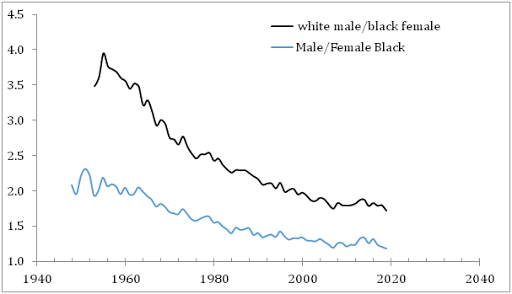## 9/29/09

### CPI components in Japan: Medical care and education

As we discussed many times in this blog, Japan has an eternal problem with deflation. The headline CPI and its components are on a long-term decline. It will continue another 40 years because the level of labor force in Japan has been and will be decreasing in line with the ageing and overall depopulation. Today, Japan Statistics released an estimate of the consumer price index for August 2009 - http://www.stat.go.jp/english/data/cpi/1581.htm.

It is instructive, that the only two components (from ten), which did not suffer annual decrease, are the medical care and education. This is in agreement with the extraordinary high rate of growth in both these components in the USA .
Despite the surging prices for medcare and education, the US is awaiting the fate of Japan - a longer deflationary period since 2012.

## 9/25/09

### Goldman Sachs on oil in 2009 and 2010

Reuters cites an updated forecast on oil demand and price just issued by GS: \$85 per barrel by the end of 2009. Currently, oil price is down to \$66-67, but it should reach \$100 in 2009.

## 9/24/09

### The Feds must bail out Lehman Brothers

There is a fundamental question on relative merits of the previous bank bailouts - which bank did deserve a bailout and how much would it really cost? Our model of stock price, developed in articles and posts, provides an additional measure of the size of bank problems - total debt. One can estimate the debt as a product of the number of shares and their market price, which was negative for the bailed out and not bailed out banks. We base our estimate on relevant stock prices described in this blog. Table 1 lists the number of stocks, their peak negative price, and the debt:

Table 1.
....................vol............ price,\$.......debt,\$
LEH...... 689,000,000.......... -40...... -2.76E+10
C ..........11,000,000,000..... -10.......-1.10E+11
AIG....... 134,000,000........ -870 ......-1.17E+11
FRE...... 648,000,000......... -40 ........-2.59E+10
FNM...... 1,110,000,000...... -45 .......-5.00E+10
(see fictitious stock price estimates for Citigroup and AIG in Figures below)

The table shows that Lehman Brothers had smaller problems than Citigroup and AIG. So, it was was easier to bail out LEH from purely mathematical and financial point of view.

Also, the joint negative price of AIG, FRE and FNM is less than 200bn. It could be some (deliberate?) miscalculation of the >1000bn help package. The toxic debt might be bigger than the total debt associated with stock prices, but there should be unaccounted positive assets.## 9/23/09

### Beyond the bankruptcy – Fannie Mae, Freddie Mac, and Lehman Brothers

Introduction
In several previous posts on bankruptcy, we have described the evolution of share prices for banks just before their bankruptcy or on their approach to negative prices: Colonial Bank (CNB), the CIT Group (CIT), American International Group (AIG), and Lehman Brothers (LEH). It was shown that the CNB sank below the edge of bankruptcy and CIT is on the brink with unclear future. AIG has been fluctuating around the waterline for a while, and LEH was definitely ready for a bankruptcy.

On the other hand, the CIT Group and AIG are bailed out, and thus, our model predicting share prices should fail to predict the artificially supported prices. Therefore, it would be instructive to estimate the fictitious evolution of share prices after the bankruptcies or alternative evolution after the bailouts. In this post we compare Lehman Brothers, Fannie Mae (FNM) and Freddie Mac (FRE). All three had a sharp fall in the second half of 2008.

Model and data
To predict share prices we use the 3-C model [1-3]: a share price, sp(t), is described with the following empirical function:

sp(t) = A*CPI1(t+t1) + B*CPI2(t+t2) + C*(t-2000) + D (1)

, where CPI1 and CPI2 are empirically determined CPI components; t is the calendar time; A, B, C, and D are empirical coefficients. Because the prices for goods and services (in the headline CPI) can lag or lead relevant share prices the time lags t1 and t2 are introduced. Both time lags can be positive or negative. In our model, we test all possible lags between 0 and +13 months. So, we chose the best from 34*33*14*14 ~ 200,000 possible models, with two extra degrees of freedom introduced by linear and free terms.

The full set includes 34 CPI components, which are tested as predictors for each share price. Among these components are major expenditure categories: the headline CPI (C), the core CPI, i.e. the headline CPI less food and energy (CC), food and beverages (F), housing (H), apparel (A), transportation (T), medical care (M), recreation (R), education (ED), communication (CO), and other goods and services (O). All components used in the model are not seasonally adjusted ones and retrieved from the Bureau of Labor Statistics.

Results
When applied to the LEH time series between July 2003 and September 2008, the 3-C model (1) gives the following empirical relationship:

LEH(t) = -10.40*FB(t-4) + 5.84*DUR (t+2) + 67.96*(t-2000) + 952; STDEV=\$4.73 (2)

, where t is the calendar time, FB(t) is the (not seasonally adjusted consumer price) index for food leading the LEH by four months, and DUR(t+2) in the index of durable goods lagging two months behind the LEH. Figure 1 compares the stock price predicted according (2) with the observed one. This model differs from that in the previous post in two aspects. First, it uses DUR instead of the personal care index (PC). This is not a fundamental change, however. Each empirical model based on two components of the CPI includes one index, which is sensitive to the change in a given share price, and one index, which expresses the overall reaction of prices for goods and services induced by the change in the share price.
Here I would like to illustrate the process on the example of isostatic compensation of ice floating in a water-filled tank. If you put some load on a piece of ice it will sink a bit deeper, but the level of water in the tank will also increase. Similarly, if some stock price increases it will affect prices of many goods and services, not only those related to the stock. There should be a component of the CPI which expresses the overall reaction the best. One can consider it as a reference component.

Then the difference between these two components maps the change in the price. Since there are many components of the CPI, which might represent the overall reaction of the CPI on the given price change, their exchange does not influence the model much. In the case of LEH, the defining component is the index for food (or food and beverages), and the reference component is the PC or DUR.

The empirical models for FNM and FER are as follows:

FNM(t) = -7.38*FB(t-8) – 8.47*R (t+3) + 42.57*(t-2000) + 2119; STDEV=\$4.42 (3)
FRE(t) = -6.07*FB(t-4) - 3.42*O (t-9) + 57.96*(t-2000) + 1917.8; STDEV=\$4.16 (4)

, where R is the index of recreation and O is the index for other goods and services in the CPI. Figures 2 and 3 compare the observed and predicted prices for FNM and FER, respectively.
All three empirical models just describe the evolution of corresponding prices using available CPI estimates. The models do not foresee stock the price evolution beyond current knowledge. However, since relevant CPI estimates are available for the last year, i.e. 11 months after the bankruptcy of Lehman Brothers, one can formally calculate the fictitious evolution of these share prices, as shown in Figures 1through 3. The LEH price demonstrates sustainable decrease between October 2008 and March 2009. Then the price regains some strength and approaches the zero line in August 2009. If bailed out in September 2008, the Lehman Brothers would start to grow in September 2009. One cannot say the same thing about AIG – it is still unstable.
The price of Fannie Mae lags behind both defining CPI components. Therefore, the prediction is possible only till April 2009. Unfortunately, the price continues its fall. There is no sign a turn. On the contrary, the price of Freddie Mac is well predictable into 2010. In March 2009, the price reached the bottom and then started to grow. It is still negative and will stay below the zero line in 2009.

Conclusion
We have predicted fictitious share prices of three companies. This prediction might be helpful for the assessment of future policy on Freddie Mac and Fannie Mae.Figure 1. Comparison of the observed and predicted LHE’s share price. The latter is obtained using (2).Figure 2. Comparison of the observed and predicted FNM’s share price. The latter is obtained using (3).Figure 3. Comparison of the observed and predicted FRE’s share price. The latter is obtained using (4).

References
 Kitov, I., Kitov, O., (2009). Predicting share price of energy companies: June-September 2009, MPRA Paper 15863, University Library of Munich, Germany, http://mpra.ub.uni-muenchen.de/15863/01/MPRA_paper_15863.pdf
 Kitov, I., Kitov, O., (2009). Modelling selected S&P 500 share prices, MPRA Paper 15862, University Library of Munich, Germany, http://mpra.ub.uni-muenchen.de/15862/01/MPRA_paper_15862.pdf
 Kitov, I., Kitov, O., (2008). Long-Term Linear Trends In Consumer Price Indices, Journal of Applied Economic Sciences, Spiru Haret University, Faculty of Financial Management and Accounting Craiova, vol. 3(2(4)_Summ), pp. 101-112.

## 9/22/09

### Predicting bankruptcy : the case of Lehman Brothers

We continue testing our model againts bancruptcy cases. Lehman Brothers presents an old but crucial case. Before we start our quantitative analysis, we have to clarify the the term "predicting" has a narrow sense of quantitative description. In other words, we do not forecast the bankruptsy, but decompose relevant stock price, sp(t), into a sum of two CPI components, a linear and a free term:

sp(t) = A*CPI1(t+t1) + B*CPI2(t+t2) + C*(t-2000) + D (1)

where CPI1 and CPI2 are defining CPI components; t is the calendar time; A, B, C, and D are empirically determined coefficients. Because prices for good and services can lag or lead relevant share prices the time lags t1 and t2 are introduced. Both time lags can be positive or negative. In our model, we test all possible lags between 0 and +13 months. So, we do not pretend that one could see the fall of LEH months before it had happened.

So, the empirical model describing the time history of LEH share price is as follows:

LEH(t) = -9.75*F(t-4) – 8.13*PC (t-2) + 94.9*(t-2000) + 2802 (2)

, where t is the calendar time, F(t) is the (not seasonally adjusted consumer price) index for food and beverages, leading the LEH(t) by four months, and PC(t-13) in the index of personal care leading the LEH(t) by 2 months. Or this model we used LEH prices between July 2003 and September 2008; the CPI components were taken till December 2008, i.e. three months ahead of the last LEH reading. Figure 1 demonstrates that the model predicted the fall below zero but three months later. Standard deviation of the residual is \$4.7.
Once again, this model could not be obtained in August 2008.Figure 1. Observed and predicted LEH share price.

### Predicting bank bankruptcy – the case of AIG

Update 1. There is a post at MarketBeat devoted to AIG : AIG Shares: Still Not Worth Anything. In general it supports our model with a negative estimate of the share price. The case is getting more and more exiting ...
In the previous post on bankruptcy, we have described the evolution of share prices for the Colonial Bank (CNB) and the CIT Group (CIT). It was shown that CNB sank below the edge of bankruptcy. (All share prices are retrieved from YahooFinance.) Here we investigate the case of AIG. It has been bailed out. Not considering political, economic and financial aftermaths of such a bankruptcy we focus on the prediction by our 3-C model: a share price, sp(t), is described with the following empirical function:

sp(t) = A*CPI1(t+t1) + B*CPI2(t+t2) + C*(t-2000) + D (1)

where CPI1 and CPI2 are defining CPI components; t is the calendar time; A, B, C, and D are empirically determined coefficients. Because prices for good and services can lag or lead relevant share prices the time lags t1 and t2 are introduced. Both time lags can be positive or negative. In our model, we test all possible lags between 0 and +13 months. So, we chose the best from 34*33*14*14 ~ 200,000 possible models, with two extra degrees of freedom introduced by linear and free terms.

The full set includes 34 CPI components, which are tested as predictors for each share price. Among these components are major expenditure categories: the headline CPI (C), the core CPI, i.e. the headline CPI less food and energy (CC), food and beverages (F), housing (H), apparel (A), transportation (T), medical care (M), recreation (R), education (ED), communication (CO), and other goods and services (O). All components used in the model are not seasonally adjusted ones and retrieved from the Bureau of Labor Statistics.
When applied to AIG time series between July 2003 and August 2009, the 3-C model (1) for AIG is as follows:

AIG(t) = -89.29*F(t) – 08.31*FB (t-13) + 1031*(t-2000) + 32666 (2)

, where t is the calendar time, F(t) is the (not seasonally adjusted consumer price) index for food and beverages and FB(t-13) in the index of food without beverages leading AIG(t) by 13 months.
Figure 1 compares the stock price predicted according (2) with the observed one. There are two dives of the predicted price below zero – March and August 2009, the latter being of -\$123. With standard deviation of \$102, the predicted price in August likely hints at unresolved problems with AIG. However, the negative price might also result from the uncertainty in F and FB measurements.
We will continue tracking AIG stock price and reporting our predictions.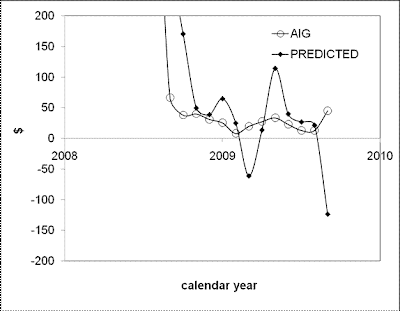Figure 1. Comparison of the observed and predicted AIG’s share price. The latter is obtained using (2).

### Share price of select energy companies. Part 2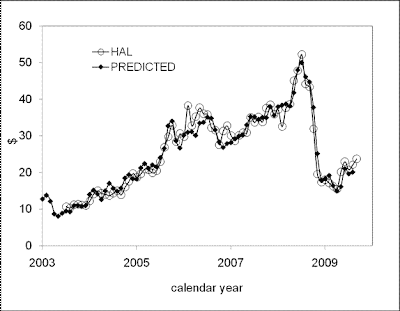Figure 12. Observed and 3-C predicted Halliburton share prices: HAL(t) = -3.66*EC(t-6) +2.42*CF(t+1) - 0.41*(t-2000) - 37, where EC is the index of education and communication and CF is the headline CPI less food. Standard deviation is \$2.35.Figure 13. Observed and 3-C predicted Hess CP share prices: HES(t) = 0.61*E(t+1) + 4.1*RENT(t-7) – 26.4*(t-2000) - 865.7, where EC is the index of education and communication and CF is the headline CPI less food. Standard deviation is \$5.92.Figure 14. Observed and 3-C predicted Massey Energy CP share prices: MEE(t) = 3.25*CSH(t+1) - 6.37*CFSHE(t-11) + 2.33*(t-2000) + 592.9, where CSH is the headline CPI less shelter and CFSHE is the headline CPI less food, shelter and energy. Standard deviation is \$6.30.Figure 15. Observed and 3-C predicted Murphy Oil CP share prices: MUR(t) = 0.83*T(t+1) – 6.25*HFO(t) + 6.20*(t-2000) + 655.9, where T is the index of transportation and HFO is the index of household furnishing and operations. Standard deviation is \$4.27.Figure 16. Observed and 3-C predicted Noble Energy share prices: NBL(t) = 0.86*T(t+2) + 2.88*RENT(t-3) - 15.8*(t-2000) - 694.4, where T is the index of transportation and RENT is the index of rent. Standard deviation is \$3.97.Figure 17. Observed and 3-C predicted National Oilwell Varco share prices: NOV(t) = 4.13*C(t+1) – 10.87*HFO(t+1) - 9.26*(t-2000) + 641.5, where C is the headline CPI and HFO is the index of household furnishing and operations. Standard deviation is \$5.28.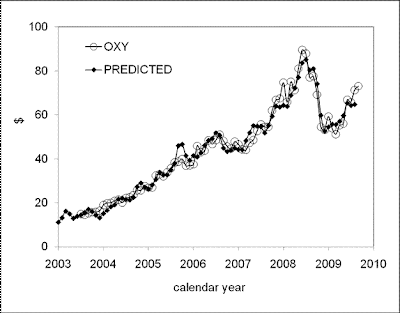Figure 18. Observed and 3-C predicted Occidental Petroleum share prices: OXY(t) = 0.73*T(t+1) + 1.17*MCS(t-5) - 12.36*(t-2000) - 405.5, where T is the index of transportation and MCS is the index of medical care services. Standard deviation is \$3.25.Figure 19. Observed and 3-C predicted Pioneer Natural Resources share prices: PXD(t) = - 3.24*EC(t-6) + 0.43*E(t+1) + 4.08*(t-2000) + 305.6, where EC is the index of education and communication and E is the index of energy. Standard deviation is \$3.73.Figure 20. Observed and 3-C predicted Rowan Companies share prices: RDC(t) = - 1.74*F(t-5) + 0.49*TPR(t+1) + 9.59*(t-2000) + 222.5, where F is the index of food and beverages and TPR is the index of private transportation. Standard deviation is \$2.85.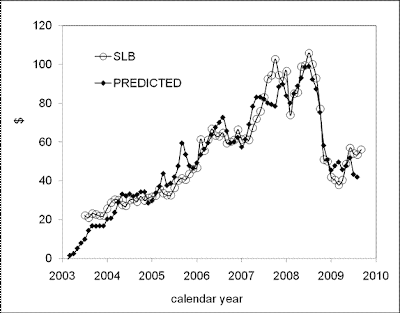Figure 21. Observed and 3-C predicted Schlumberger share prices: SLB(t) = - 5.47*F(t-11) + 0.93*T(t+1) + 36.9*(t-2000) + 695.9, where F is the index of food and beverages and T is the index of transportation. Standard deviation is \$7.24.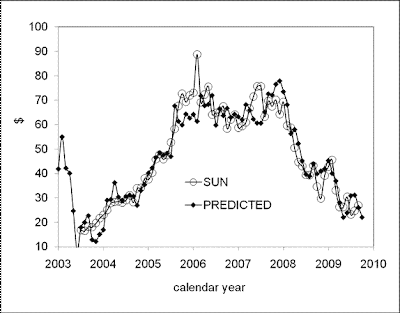Figure 22. Observed and 3-C predicted Sunoco share prices: SUN(t) = - 15.9*CO(t-8) + 10.17*HFO(t-8) - 19.8*(t-2000) + 257.7, where CO is the index of communication and HFO is the index of household furnishing and operations. Standard deviation is \$6.12.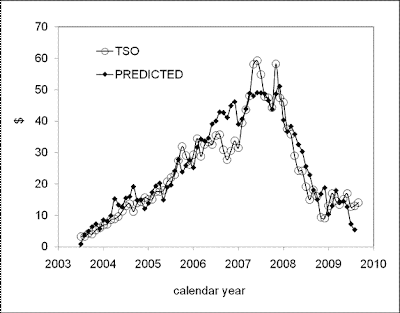Figure 23. Observed and 3-C predicted Tesoro share prices: TSO(t) = -2.85*F(t+2) - 5.44*FB(t-11) + 54.4*(t-2000) + 1273, where F is the index of food and beverages and FB is the index of food only. Standard deviation is \$6.12.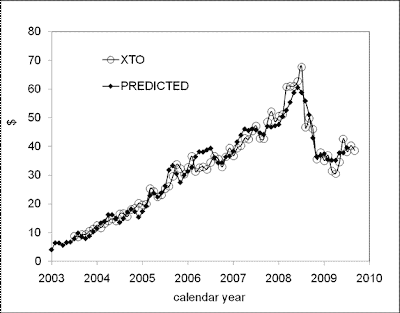Figure 24. Observed and 3-C predicted XTO Energy share prices: XTO(t) = 1.49*CSH(t+2) – 1.73*FB(t-6) + 9.87*(t-2000) + 18.1, where CSH is the headline CPI less shelter and FB is the index of food only. Standard deviation is \$3.29.

## 9/21/09

### Share price of select energy companies

Since September 16, 2009 the readings of the headline CPI and its components for August 2009 are available (we retrieve all CPI data from http://www.bls.gov/data). We recalculate our model for selected stock prices from the S&P 500 list. First was ConocoPhillips since it provides a good example of a company, which share price has been leading defining components of the CPI. Here we present several companied from the “energy” subcategory of S&P 500. It is instructive that these companies are rather different than similar in the evolution of share price and thus in defining CPI components.

In the previous posts and articles [1,2] we introduced several models with varying number of CPI components. In our view, the best one, we call it 3-C model, is seeking those two CPI components from 34 pre-selected ones, which minimize the difference between observed (monthly closing price adjusted for dividends and splits) and predicted prices for the period between July 2003 and August 2009. This model also includes free term (constant) and linear time term [3-6], which compensates well know linear (time) trends between various CPI components.

In the previous post we reported the empirical 3-C model for COP:

COP(t)= 3.41CF(t+1) - 7.17EC(t-6) + 7.22*(t-2000) + 145.76

where t is the calendar time, CF is the headline CPI less food, which lags behind COP by one months, and EC is the index for education and communication leading COP by six months. Standard deviation between the curves is \$3.96 for the period between July 2003 and August 2009. Figure 1 displays the observed and predicted prices.Figure 1. Observed and 3-C predicted COP’s share price.

Below we present analytic models and figures for several energy companies without comments. One can judge the accuracy of the predictions and the similarity/difference between the companies.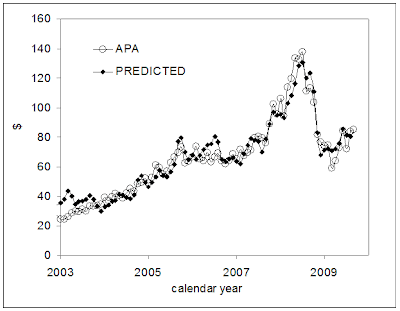Figure 2. Observed and 3-C predicted Apache Corp.’s share price: APA(t) = 1.35*TPR(t+1) + 3.21*MCS(t-7) + 47.20*(t-2000) - 973, where TPR is the index of private transportation, MCS is the index of medical care services. Standard deviation is \$6.36.Figure 3. Observed and 3-C predicted Anadarko Petroleum Corp.’s share price: APC(t) = 3.31*EC(t+2) – 1.74*CSHF(t-5) + 5.49*(t-2000) + 58, where EC is the index of education and transportation, CSHF is the headline CPI less shelter and food. Standard deviation is \$3.22.Figure 4. Observed and 3-C predicted Baker Hughes’ share price: BHI(t) = 4.93*F(t-5) – 0.79*T(t+1) + 32.53*(t-2000) + 662, where F is the index of food and beverages, T is the index of transportation. Standard deviation is \$5.30.Figure 5. Observed and 3-C predicted Peabody Energy Corp.’s share price: BTU(t) = 0.64*FU(t-4) + 1.36*TPR(t+1) – 8.37*(t-2000) - 266, where FU is the index of (housing) fuels and utilities, TPR is the index of private transportation. Standard deviation is \$4.35.Figure 6. Observed and 3-C predicted Cameron Intl. CP share prices CAM(t) = 0.64*TPR(t+1) + 2.65*RENT(t-4) - 17.1*(t-2000) - 610, where FU is the index of (housing) fuels and utilities, TPR is the index of private transportation. Standard deviation is \$3.38.Figure 7. Observed and 3-C predicted Chesapeake Energy Corporation share price: CHK(t) = 0.41*E(t+1) - 1.50*ED(t-7) - 12.1*(t-2000) + 102.7, where E is the index of energy and ED is the index of education. Standard deviation is \$2.70.Figure 8. Observed and 3-C predicted Consol Energy share price: CNX(t) = 6.11*C(t+2) – 2.62*ED(t-5) – 2.81*(t-2000) - 767.5, where C is the headline CPI and ED is the index of education. Standard deviation is \$5.65.Figure 9. Observed and 3-C predicted Chevron share price: CVX(t) = 0.25*E(t+1) + 2.76*MCS(t+4) – 37.6*(t-2000) - 716.1, where E is the index of energy and MCS is the index of medical care services. Standard deviation is \$3.26.Figure 10. Observed and 3-C predicted Devon Energy Corp.’s share price: DVN(t) = 0.79*T(t-3) + 0.46*E(t+2) – 0.68*(t-2000) - 162.7, where E is the index of energy and T is the index of transportation. Standard deviation is \$5.67.Figure 11. Observed and 3-C predicted Ensco share prices: ESV(t) = 2.23*CSH(t+1) - 2.61*FB(t-5) + 8.89*(t-2000) + 65.9, where CSH is the headline CPI less shelter and FB is the index of food only. Standard deviation is \$3.37.

References
 Kitov, I., Kitov, O., (2009). Predicting share price of energy companies: June-September 2009, MPRA Paper 15863, University Library of Munich, Germany, http://mpra.ub.uni-muenchen.de/15863/01/MPRA_paper_15863.pdf
 Kitov, I., Kitov, O., (2009). Modelling selected S&P 500 share prices, MPRA Paper 15862, University Library of Munich, Germany, http://mpra.ub.uni-muenchen.de/15862/01/MPRA_paper_15862.pdf
 Kitov, I., Kitov, O., (2008). Long-Term Linear Trends In Consumer Price Indices, Journal of Applied Economic Sciences, Spiru Haret University, Faculty of Financial Management and Accounting Craiova, vol. 3(2(4)_Summ), pp. 101-112.
 Kitov, I., (2009). Apples and oranges: relative growth rate of consumer price indices, MPRA Paper 13587, University Library of Munich, Germany, http://mpra.ub.uni-muenchen.de/13587/01/MPRA_paper_13587.pdf
 Kitov, I., Kitov, O., (2009). A fair price for motor fuel in the United States, MPRA Paper 15039, University Library of Munich, Germany, http://mpra.ub.uni-muenchen.de/15039/01/MPRA_paper_15039.pdf
 Kitov, I., Kitov, O., (2009). Sustainable trends in producer price indices, MPRA Paper 15194, University Library of Munich, Germany, http://mpra.ub.uni-muenchen.de/15194/01/MPRA_paper_15194.pdf

### Predicting bank bankruptcy: Colonial Bank vs. CIT Group Inc.

We have described the evolution of a number of share prices for S&P 500 companies using empirical relationship between the prices and the differences between various CPI components [1-3], [Seeking Alpha posts]: Bank of America (BAC) and Goldman Sachs (GS, both financials), Microsoft (MSFT, information technology), and Alcoa (AA, materials), Boeing (BA, industrials), Exxon mobile (XOM, energy), ConocoPhillips (COP, energy), etc. All these companies were described with a reasonable accuracy considering the uncertainty associated with the monthly estimates of CPI expenditure subcategories and slightly stochastic character of the monthly closing price (adjusted for dividends and splits) as a representative of corresponding share price. All in all, the concept has demonstrated a high level of consistency.

This time our task is a more exciting and challenging one. Can our model describe the approach to bankruptcy? For a regular investor this question is of the top priority. The history of dramatic decline in share prices of financial companies in 2008 and 2009 dictates an inevitable choice – comparison of the Colonial Bank (CNB) and CIT Group (CIT), which both were beyond the edge of bankruptcy but with different outcome. In this study, all share prices are retrieved from YahooFinance.

Previously, we introduced and tested several models with varying number of defining CPI components. It was found that the best model includes two defining CPI components, free term (constant) and linear time term [4-6], which compensates well known the linear trends between the defining CPI components. For historical reasons we call it 3-C model. In this study, we are seeking for those two CPI components from a full set of components, which minimize the (RMS) difference between observed and predicted prices.

The 3-C model for a share price sp(t) is as follows:

sp(t) = A*CPI1(t+t1) + B*CPI2(t+t2) + C*(t-2000) + D (1)

where CPI1 and CPI2 are defining CPI components; t is the calendar time; A, B, C, and D are empirically determined coefficients. Because prices for good and services can lag or lead relevant share prices the time lags t1 and t2 are introduced. Both time lags can be positive or negative. In our model, we test all possible lags between 0 and +13 months. So, we chose the best from 34*33*14*14 ~ 200,000 possible models, with two extra degrees of freedom introduced by linear and free terms.

The full set includes 34 CPI components, which are tested as predictors for each share price. Among these components are major expenditure categories: the headline CPI (C), the core CPI, i.e. the headline CPI less food and energy (CC), food and beverages (F), housing (H), apparel (A), transportation (T), medical care (M), recreation (R), education (ED), communication (CO), and other goods and services (O). All components used in the model are not seasonally adjusted ones and retrieved from the Bureau of Labor Statistics.

In  we have revealed and explained the presence of linear trends in the differences between various components of the CPI. It was also found that the trends exist during long but finite periods and then their slops turn to opposite signs. Accordingly, the evolution of the differences between CPI components since 1960 can be approximated by a piece-wise linear function. When the trends turn, the coefficients in the link between CPI and share price also change. Because of this constraint we limit our modeling to the period between July 2003 and July 2009. The early date is a conservative estimate of the start of the current trend, and the latter one corresponds to the bankruptcy of Colonial Bank.

Here, we would like to emphasise that the usage of the differences instead of CPI components is caused by two crucial factors. First, we presume that the evolution of a share price depends only on the pricing power of goods and services associated with the company relative to other goods and services. Then, the difference in pricing powers of two companies should be directly mapped into the difference in the growth in relevant consumer price indices. Therefore, we assume that there exists a pair of CPI components, which reflect the best relative evolution of the share prices. Such a pair might not be always available among eight major expenditure categories. As a result, one needs two or more differences between CPI components to accurately predict the evolution of a share price.

Second factor consists in the presence of sustainable (linear and nonlinear) trends in the difference. This is the phenomenon we have described in [4-6]. Effectively, one can accurately decompose any share price using several CPI components. However, such decomposition does not allow forecasting without knowledge of the evolution of relevant CPI subcategories. The trends in the differences make it possible to predict at longer time horizons.

Now we apply the 3-C model (1) to the CNB’s and CIT Group’s share prices. The (LSQR) best-fit empirical relationship is as follows:

CNB(t) = 1.58*F(t) – 1.39*O (t-10) + 20.61*(t-2000) + 633 (2)
CIT(t) = -4.14*F(t-6) – 3.10*FB (t+4) + 43.18*(t-2000) + 1776 (3)

Empirical relationship (2) implies that CNB’s share price evolve in sync with the index of food and beverages (F), but lags behind the index for other goods and services (O) 10 months. Therefore, the share price depends on past and contemporary values of the defining CPI components. Effectively, it allows predicting CNB price only in the past. Figure 1 displays the observed and predicted price as well as their residual.

Empirical relationship (3) shows that CIT’s share price leads by six months the index of food and beverages (F) and lags four months behind the index for food only (FB). Therefore, the share price depends on past and future values of the defining CPI components. Effectively, it allows predicting CIT price only in the past.

Comparing the time histories of CNB and CIT one can conclude that the former sank right below the zero price and thus this bank deserved the bankruptcy. CIT has been closing the negative zone but still is slightly above it. The next several readings of FB will answer the question – Was it worth to bail out CIT Group? In other words, will the predicted price sink below the zero line?Figure 1. Upper panel: Comparison of the observed and predicted CNB’s share price. The latter is obtained using (2). Lower panel: the difference between the observed and predicted price.Figure 2. Upper panel: Comparison of the observed and predicted CIT’s (adjusted for dividends and splits) share price. The latter is obtained using (3). Lower panel: the difference between the observed and predicted price.

References
 Kitov, I., (2009). ConocoPhillips and Exxon Mobil stock price, Journal of Applied Research in Finances, v. 1, issue 2 (in press).
 Kitov, I., Kitov, O., (2009). Predicting share price of energy companies: June-September 2009, MPRA Paper 15863, University Library of Munich, Germany.
 Kitov, I., Kitov, O., (2009). Modelling selected S&P 500 share prices, MPRA Paper 15862, University Library of Munich, Germany
 Kitov, I., Kitov, O., (2008). Long-Term Linear Trends In Consumer Price Indices, Journal of Applied Economic Sciences, Spiru Haret University, Faculty of Financial Management and Accounting Craiova, vol. 3(2(4)_Summ), pp. 101-112.
 Kitov, I., Kitov, O., (2009a). A fair price for motor fuel in the United States, MPRA Paper 15039, University Library of Munich, Germany
 Kitov, I., Kitov, O., (2009b). Sustainable trends in producer price indices, Journal of Applied Research in Finance, v. 1, issue 1

## 9/20/09

### The Phillips curve regains attention

Laurel Graefe, senior economic research analyst at the Atlanta Fed, wrote a short post on the Phillips curve for macroblog. The evolution of unemployment and inflation in the USA since 2008 actually needs a very detailed analysis.

I agree that the Phillips curve is not a productive idea since it does not explain observations in many countries. Moreover it is counterproductive because unemployment in the USA (and some other developed countries) lags behind inflation. We have published a number of papers on this issue. For example,"The Anti-Phillips curve" . It is funny, that Laurel being a Senior Economic Research Analyst does share our opinion on the helplessness of the conventional Phillips curve.

Also, we fully agreed that it is the best time to make deciding study of the link between inflation and unemployment - the change in both variables is fast and dramatic. Our generalized model, linking both inflation and unemployment to the change in the level of labor force, should also be tested against the most recent data. Usually, we do not use high-frequency data for our analysis because the uncertainty of the change over short periods is too high, but the rate of growth in unemployment and fall in inflation is really large to overcome this shortcoming.
We are in the initial stage of the analysis and will trace the near future developments. At this stage, the generalized model looks an adequate one - the drop in CPI fully compensates the rise in unemployment according to their coefficients in the relationship. In other words, the change in unemployment is driven by the change in inflation.
On the other hand, the time lag suddenly fell from 2.5 years to several months in 2008. We need more time to understand the causes of the shift. It is not excluded that the current state is related to panic deviation from the generalized equilibrium. Then the spike in unemployment and inflation are only a short one fluctuation.

## 9/17/09

### CPI vs. core CPI: August 2009

Here we revisit our previous analysis of the difference between headline and core CPI in the USA . Figures 1 and 2 illustrate the evolution of the difference which is apparently characterized by the presence of three distinct segments with linear trend and two short periods of change in the trends. Between 1960 and 1978, the difference is relatively stable and varies in a narrow range around +1 unit of index. Between 1979 and 1982, the curve falls to -2 and then suddenly changes its direction from downward to upward one.
Between 1982 and 1999, the core CPI was growing consistently faster and a gap of about 10 units was created through 1999. The curve between 1982 and 1999 is best represented by a straight line with small-amplitude deviations, the latter likely associated with measurement noise. The slope of the linear regression line presented in Figure 2 is -0.67 with R2=0.96. In other words, during these eighteen years the headline CPI was growing by 0.67 units of index faster than the core CPI.
If to assume that the evolution of both indices is driven by independent stochastic process like random walks, then the deviation between the indices would be a stochastic process itself. From Figure 2, it is more reasonable and reliable to assume, however, that there is a tight link between these variables, which provides the observed linear growth of the difference between them. For purely stochastic and independent variables such a linear behavior is highly unlikely. Because the CPI inflation is predefined by the labor force growth rate (Kitov, 2006ab) the evolution of the core CPI is also predefined.
There was another period of high volatility between 1999 and 2003 similar to that observed between 1979 and 1982. Between 2003 and 2008, the gap between the core CPI and CPI was closing in line with a faster growing CPI. In , we extrapolated the rate of convergence between the CPI and core CPI observed after 2003, as displayed in Figure 3, one can estimate the intercept time somewhere between 2009 and 2010. This linear trend of convergence is very robust with R2=0.86, as was the divergence trend between 1982 and 1999. The convergence is faster - approximately 1.6 units of index per year. This prediction was accurate in terms of the turn to a new positive trend but timing was slightly wrong. The turn started in the middle of 2008 with a severe fall in the difference down to -4. This early start of the transition to the new trend was accompanied by extraordinary volatility. After the difference reached the bottom at -4, it quickly (during six months) rebounded to +6. At this point we made (in this blog and several articles [2-4]) a new assumption that the difference would be freely falling since March 2009. Such behavior has been actually observed, as Figure 4 demonstrates. The return to the new linear trend may include a slight overshoot below the trend line in 2009 or early 2010.
What will happen after 2010? It is likely that the difference will fluctuate around the new trend, which characteristics have to be established yet.Figure 1. The difference between the core CPI and headline CPI: update from October 2007 to August 2009.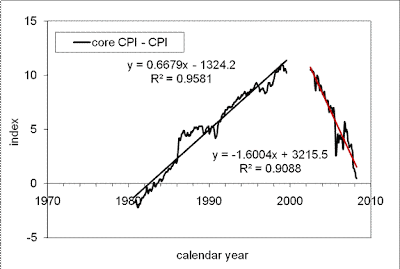Figure 2. Linear regression of the difference between the core CPI and CPI.Figure 3. Extrapolation of the trend in the differnce between core CPI and CPI beyond 2007 .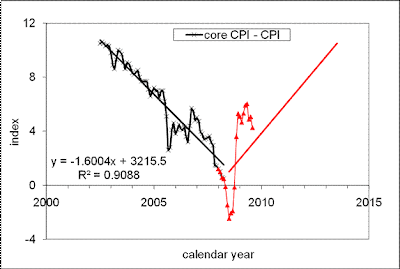Figure 4. The return to the new linear trend. An overshoot below the trend line may happen in 2009 or early 2010. Then, the differnce will align around the new trend, which will emerge promptly.

References
 Kitov, I., Kitov, O., (2008). Long-Term Linear Trends In Consumer Price Indices, Journal of Applied Economic Sciences, Spiru Haret University, Faculty of Financial Management and Accounting Craiova, vol. III(2(4)_Summ), pp. 101-112. http://www.jaes.reprograph.ro/articles/Kitov.pdf

 Kitov, I., Kitov, O., (2009). A fair price for motor fuel in the United States, MPRA Paper 15039, University Library of Munich, Germany, http://mpra.ub.uni-muenchen.de/15039/01/MPRA_paper_15039.pdf

 Kitov, I., Kitov, O., (2009). Apples and oranges: relative growth rate of consumer price indices, MPRA Paper 13587, University Library of Munich, Germany, http://mpra.ub.uni-muenchen.de/13587/,
http://mpra.ub.uni-muenchen.de/13587/01/MPRA_paper_13587.pdf

 Kitov, I., Kitov, O., (2009). Sustainable trends in producer price indices, MPRA Paper 15194, University Library of Munich, Germany, http://mpra.ub.uni-muenchen.de/15194/01/MPRA_paper_15194.pdf

### Price inflation: Q&A

Sometimes Q&A is the best way to present new concepts. I expect this post to grow over time and welcome questions related to theoretical and empirical aspects of price inflation in developed countries. Emerging and developing countries are also of interest, especially the cases of hyperinflation.

Q1. What is it - price inflation?
In narrow sense, price inflation is a reaction of economic system to changing level of labor force. Inflation is the process which recovers personal income distribution to its natural and fixed (rigid) shape. As a monetary process, inflation is expressed in changing prices for goods and services (G&S), with the total cost of all these G&S exactly determined by real economic growth, if any, and inflation, as predefined by the change in labor force level.
In developed (capitalist) economies, prices for G&S have to interact and compete, i.e. to be governed by "invisible hand", in order to find some final (but not predetermined!) configuration, which corresponds to the total nominal cost of the G&S. This total cost (nominal GDP) is defined by real economic growth and inflation.
(Detailed explanation of price inflation as an economic phenomenon and process as well as relevant quantitative analysis are given in our papers.)

Q2. Is it possible to describe the link between inflation and labor force change using simple mathematical representation?
There exists a linear lagged relationship between inflation, π(t), unemployment, UE(t), and the change rate of labor force, dLF(t)/LF(t):

π(t+t1)= AdLF(t)/LF(t) + BUE(t+t2) + C

where A, B, and C are country-specific empirical constant (this relationship is normalized to inflation); t1 and t2 are time lags between the change in labor force and the reaction of inflation and unemployment. These lags may reach several years and there is no general rule which variable reacts first.

Q3. Is inflation an economic ill, constantly threatening economic stability?
There is no statistical or any quantitative link between inflation and real economic growth except the one, which is related to population changes. There is a formal statistical and econometric prove available in our papers that real economic growth depends only on the attained level of real GDP per capita and the change rate of population of a country-specific age. This defining age is nine years in the USA.

Q4. Is deflation harmful?
Since real economic growth and inflation are not directly related, the recession and deflation (like observed in Japan) have different sources. For example, the former process is approaching its end in Japan and real economic growth (per capita) is on its potential level (http://inflationusa.blogspot.com/2007/07/modelling-evolution-of-real-gdp-in.html). The latter process will last the next 40 years with increasing amplitude (http://inflationusa.blogspot.com/2007/08/prediction-of-cpi-inflation-in-japan.html )

Q5. Is inflation unpredictable?
Inflation can be accurately predicted at various time horizons in developed countries. Examples are the USA, Japan, France, Germany, Canada, Austria, and the UK. The accuracy of inflation prediction is completely defined by the accuracy of labor force measurements, as follows from the relationship in Q2. There are some simple means to improve current labor force estimates.

Q6. Do central bankers control inflation and inflationary expectations through clear inflation targeting?
Because inflation is completely defined by the change rate of labor force Central Banks do not control inflation as it is. They can potentially control the balance between inflation and unemployment, which are linear lagged function of the change rate of labor force.
Quantitative prediction of the history of inflation in USA is a good example that the Fed does not control inflation. The history of inflation in France demonstrates that the suppression of money supply results in elevated unemployment. So, this is an example of negative affect of central bank in sense of high unemployment balancing low inflation.

## 9/16/09

### ConocoPhillips share price revisited

Since September 16, the readings of the headline CPI and its components for August 2009 are available (we retrieve all CPI data from http://www.bls.gov/data). We recalculate our model for selected stock prices from the S&P 500 list. First is ConocoPhillips since it provides a good example of a company, which share price has been leading defining components of the CPI. In the previous posts and articles [1,2] we introduced several models with varying number of CPI components. Here we compare two models. The first one, we call it 3-C model, is seeking those two CPI components from 34 pre-selected ones, which minimize the difference between observed (monthly closing price adjusted for dividends and splits) and predicted prices for the period between July 2003 and August 2009. This model also includes free term (constant) and linear time term [3-6], which compensates well know linear (time) trends between various CPI components. The second model (5-C) uses four CPI components and free term. The number of potential defining components is reduced to ten: food (F), housing (H), apparel (A), transportations (T), medical care (M), recreation (R), education and communication (EC), other goods and services (O), the headline CPI (C), and the core CPI (CC). These components compile a full set of the CPI expenditure categories, and also comprise a subset of the 34-component set.
The 3-C model for COP is as follows:

COP(t)= 3.41CF(t+1) - 7.17EC(t-6) + 7.22*(t-2000) + 145.76 (1)

where CF is the headline CPI less food. It does not differ from the model presented in the previous post except the time shifts are +1 (was +2) month and -6 (was -5) months now. This is due to the inclusion on the CPI readings for August. Figure 1 shows the predicted and observed curves. Standard deviation between the curves is \$3.96 for the period between July 2003 and August 2009.Figure 1. Observed and 3-C predicted COP’s share prices.

The 5-C model does not include the linear time term, but two components extra to the 3-C model play this role. The best 5-C model also shows that the stock price leads all defining CPI components:

COP(t)= 1.53H(t+3) – 8.29R(t+1) – 4.90EC(t-6) + 3.53C(t+1) + 518.4 (2)

There components from the four defining lag behind COP share price and only the EC leads by six months. Figure 2 presents the model. Standard deviation between the curves is only \$3.03 for the period between July 2003 and August 2009, which is better than the accuracy of the 3-C model.Figure 2. Observed and 5-C predicted COP’s share prices.

At first glance, the 5-C prediction is better that the 3-C one. However, this conclusion is not fully correct. As mentioned above, the extra two components chiefly represent the linear trend. Figure 2 illustrates the phenomenon. The difference between R and EC actually provides a linear trend with small fluctuations, which slightly suppress the residual of the 3-C model. Among all pairs of CPI components -8.29R(t+1) – 4.90EC(t-6) suppresses the model residual (noise) the most efficient. It is a positive side of the 5-C model. It has a negative side as well. The difference may diverge from the linear trend in the next several months and will increase the residual and thus the accuracy of prediction instead of suppression. So, we do prefer using the 3-C model for the prediction of share prices. Figure 4 depicts both residuals.Figure 3. Two differences between defining components in (2).

We will continue updating both empirical models. The next obvious date is October 1, 2009 with the next closing share price for September.
References
 Kitov, I., Kitov, O., (2009). Predicting share price of energy companies: June-September 2009, MPRA Paper 15863, University Library of Munich, Germany, http://mpra.ub.uni-muenchen.de/15863/01/MPRA_paper_15863.pdf
 Kitov, I., Kitov, O., (2009). Modelling selected S&P 500 share prices, MPRA Paper 15862, University Library of Munich, Germany, http://mpra.ub.uni-muenchen.de/15862/01/MPRA_paper_15862.pdf
 Kitov, I., Kitov, O., (2008). Long-Term Linear Trends In Consumer Price Indices, Journal of Applied Economic Sciences, Spiru Haret University, Faculty of Financial Management and Accounting Craiova, vol. 3(2(4)_Summ), pp. 101-112.
 Kitov, I., (2009). Apples and oranges: relative growth rate of consumer price indices, MPRA Paper 13587, University Library of Munich, Germany, http://mpra.ub.uni-muenchen.de/13587/01/MPRA_paper_13587.pdf
 Kitov, I., Kitov, O., (2009). A fair price for motor fuel in the United States, MPRA Paper 15039, University Library of Munich, Germany, http://mpra.ub.uni-muenchen.de/15039/01/MPRA_paper_15039.pdf
 Kitov, I., Kitov, O., (2009). Sustainable trends in producer price indices, MPRA Paper 15194, University Library of Munich, Germany, http://mpra.ub.uni-muenchen.de/15194/01/MPRA_paper_15194.pdf

### Predicting Microsoft stock price

We continue modelling various stock prices. This post is devoted to Microsoft (MSFT). In my article on the prediction of stock prices for COP and XOM - “Predicting ConocoPhillips and Exxon Mobil stock price”, Journal of Applied Research in Finance, v.2, 2009 (a draft version is available here.), only a very crude model of the stock price evolution was presented. Now a more reliable model is available in the following form:

sp(t)= A1S1(t+t1) + A2S2(t+t2) + A3t +A4

where sp(t) is the stock price at time t, A1 through A4 are empirical coefficients, S1 and S2 are components of headline CPI, t1 and t2 are time difference (negative or positive) between the change in the stock price and relevant changes in the CPI components. Term A3t is introduced to compensate linear trends observed in the difference between the components.

For Microsoft, an accurate empirical model is as follows:

MSFT(t)= -3.61*R(t-1) – 1.22*MSC(t-11) + 23.5*(t-2000) + 680.5.

where t is the calendar time, R(t-1) is the index for recreation leading MSFT(t) by one month, MCS(t-11) is the index for medical service leading the MSFT(t) by eleven months. The linear trend term 23.5*(t-2000) compensates the trend term in the difference between R and MCS. Standard deviation between the curves is \$1.75 for the period between July 2003 and August 2009.Figure 1. Comparison of measured (open circles) and predicted (solid diamonds) stock prices of Microsoft

### Unemployment in Germany at 11% in 2011

Original paper:
Kitov, I., (2007). Exact prediction of inflation and unemployment in Germany, MPRA Paper 5088, University Library of Munich, Germany, http://ideas.repec.org/p/pra/mprapa/5088.html
On July 1, we presented a model of the evolution of unemployment in Germany developed in . There were new readings of unemployment (from 2005 to 2007) and labor force (from 2004 to 2006) available at the OECD , and we were able to verify the model, to extend it in time, and to predict beyond 2009.
The model stats, that unemployment in developed countries is a function of labor force change [2-5]. There is a general expectation of a good fit between these two variables for Germany. Figure 1 repeats the results of a simple manual trial-and-error process for the period between 1965 and 2007. Since such procedure is based on visual fit only, no statistical estimates of the residual were made.
The resulting relationship between unemployment and labor force in Germany is as follows:
UE(t) = 2.5*dLF(t-5)/LF(t-5) + 0.04, before 1995
UE(t) = 2.5*dLF(t-5)/LF(t-5) + 0.08, after 1995 (1)

where UE(t) is the rate of unemployment in Germany at time t, LF(t-5)is the level of labor force five years earlier. The observed unemployment needs a structural break to be introduced in 1995, which is easily explained by the reunification, i.e. 1990 plus the five-year lag. Free term in (1) underwent a significant increase, but the slope of 2.5 and the time lag of 5 years did not change, however. (It should be noted that the slope depends of definition of unemployment and labor force, i.e. on reporting agency.) One can read (1) in the following way – a 1% increase in labor force in Germany results in 2.5 % increase in unemployment five years later. When the labor force does not change, the level of unemployment is constant at 8%.
An important finding here is that unemployment in Germany increases with increasing rate of labor force growth. So, the remedy against high unemployment in Germany consists in the (likely artificial) reduction of labor force growth. The estimates of unemployment are provided by the OECD and cover the period till 2007. In 2008, the unemployment had to reach the bottom around 8% (notice that this prediction was made in 2004!). In 2009, the rate of unemployment in Germany should be increasing due to the increase in labor force five years ago, not due to the crisis. In 2010, it will reach its peak value at 11% and then will start to decline into the 2010s.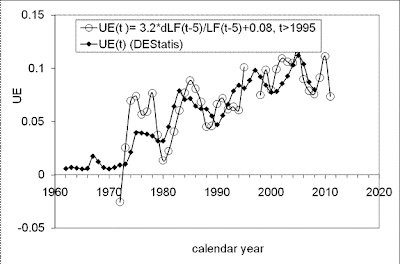Figure 1. Observed and predicted rate of unemployment in Germany.

In order to test our model, we have borrowed unemployment estimates from the DEStatics (Federal Statistics Office). They are slightly different from those provided by the OECD, and are issued at monthly frequency. Figure 2 presents the measured and predicted unemployment rate for the period between 2000 and 2012. The predicted curve has slightly higher coefficient than that obtained from the OECD data (+3.2):

UE(t) = 3.2*dLF(t-5)/LF(t-5) + 0.08 (2),

but the time shift is the same – five years. All in all, both curves are very close. Therefore, our prediction of 11% rate in 2011 is a reliable one. Germany will suffer another surge in unemployment rate during the next 18 months. In 2010 the rate will reach 9%.Figure 2. Observed and predicted rate of unemployment in Germany - likely to reach 11% in 2011.

References
 Kitov, I., (2007). Exact prediction of inflation and unemployment in Germany, MPRA Paper 5088, University Library of Munich, Germany, http://ideas.repec.org/p/pra/mprapa/5088.html
 Kitov, I., Kitov, O., Dolinskaya, S., (2007). Inflation as a function of labor force change rate: cointegration test for the USA, MPRA Paper 2734, University Library of Munich, Germany, ideas.repec.org/p/pra/mprapa/2734.html
 Kitov, I., (2007). Inflation, Unemployment, Labor Force Change in European countries, in T. Nagakawa (Ed.), Business Fluctuations and Cycles, pp. 67-112, Hauppauge NY: Nova Science Publishers
 Kitov, I., (2007). Exact prediction of inflation and unemployment in Canada, MPRA Paper 5015, University Library of Munich, Germany, http://ideas.repec.org/p/pra/mprapa/5015.html
 Kitov, I., Kitov, O., (2009). Unemployment and inflation in Western Europe: solution by the boundary element method, MPRA Paper 14341, University Library of Munich, Germany

### Krugman, Cochrane, Altig ...

There is an active discussion of the future of economics profession. Where should we ( e.g. the broader scientific community) seek the ideas and tools, which could save economics as an emergent science?
Some mainstream economists like Krugman, Cochrane, Altig and others again discuss these problems in light of which school of economic thought should prevail. I guess that this discussion will stretch straight into the next failure to predict significant economic phenomenon; like deflation in the USA starting in 2012. So, despite its activity the discussion seems to be a hopeless one.

We deserve a better treat. Therefore, the scientific community must formulate clear questions and set some threshold requirements for economics as a science to be allowed to shape and control economic and social life. In a sense, we are all customers of economic theory because it ifluences in one way or another the decisions made by economic and financial authorities. As the customers we should ask the economic profession to formulate a new research plan (not to argue which school is wrong). This plan has to define clear (for general public and experts in various fields) ideas and tools which are necessary to answer the question why the theory has failed to describe 2007-2010, and when it expects a new unpredictable change likely to happen. Meanwhile, it would be helpful for economists to regain public trust. This current discussion on the difference between various (failed) approaches does not look like helpful. If they follow the route of the negation of the presence of educated audience waiting for reasonable answers, they will completely detach themselves from the scientific community and general public as well.

### PPI of copper ores and grains: update. July and August 2009

Lately, we have demonstrated that the evolution of various components of CPI and PPI in the United States is not a random process but rather a predetermined one with long-term sustainable trends [1-4]. Using these trends, one can predict consumer and producer price indices for various goods, services and commodities [5-7]. Moreover, share prices for selected S&P 500 companies are also well described in the past by the differences in the PPI and CPI [8,9]. The near future will test the predictive power of our model.
In [4-7], we presented the evolution many commodities with varying weight in the PPI. But there are many more commodities of interest for producers, consumers and investors. In this article, we present the producer price indices of copper and grain. The evolution of the indices of these two commodities is independent, but both give a good example of the absence of clear sustainable trends. In other words, not every commodity price is predictable as an extension of a liner trend, as mentioned in . However, in the short-term, there is observed some inertia in the evolution of both prices. Therefore, one can predict the indices at a several month horizon. On July 27, we published selected results of our study for June 2009. This is a bi-monthly update, which includes two new readings for July and August 2009. Both commodities demonstrate continuation of short-term trends. Supposedly, these trends survive till the end of 2009.
Figure 1 displays the overall (commodity) PPI and the index of copper ores since 1988. The difference of these two indices has a remarkable history; no big change between 1988 and 2005, and then a sudden giant jump in the copper index. Fluctuations between 350 and 500 points lasted three years, and then the index dropped by ~300 units back to the PPI level. Despite the early start in 2005 the growth seems to be oil independent, the most recent fall looks to be driven by oil price and the overall economic slowdown. In July and August 2009, one can observe a sustainable increase in the cooper index likely associated with the rise in oil price. In the long-run, oil price should decline to the level of ~\$25 in 2016. Therefore, copper price will likely not be growing to its peak in April 2008 (491.7), but will likely return to heights around 350. (Our model based on the presence of sustainable trends in the difference between the PPI and individual PPI is not applicable to copper ores.)Figure 1. Evolution of the price index of copper ores and the PPI.

The producer price index for grains presents another difficult case. Figure 2 depicts the PPI and the index, and their difference between 1960 and 2009. There is no sustainable trend in the difference. Between 1974 and 2005, the difference demonstrated an overall growth with several spikes, the strongest one in 1996. The presence of a long-term positive trend in the difference is completely due to the growth in the PPI because the index of grains fluctuates around a constant level just slightly above 100 points. Lately, the grains index suffered the biggest rise and fall in absolute terms. The main increase started earlier in 2007 and stretched into 2008, with the peak in June 2008. Volatility of the difference during the past two years was so high that it is difficult to predict the next move of the price index of grains at a several year horizon. Seemingly, it has been repeating the trajectory of the index for crude oil in 2008 and 2009. If it is the case, one can expect that the index for grains will continue to oscillate around the constant level of ~100.

It is instructive to compare two major spikes in the grains index in 1996 and 2008 relative to the PPI. In order to avoid comparing absolute values, which undergo secular growth, the evolution of the difference between the PPI and the price index of grains normalized to the PPI. Figure 3 presents the normalized curves. The left panel shows that the spike in the grains PPI in July 1996 is similar in relative terms to that observed in 2008. The right panel tests this hypothesis: the spikes are synchronized - for the black line is shifted forward by 142 months. From this comparison, it is likely that decline in the grains index relative to the PPI will extend into the 2010s.Figure 3. Evolution of the difference between the PPI and the price index of grains normalized to the PPI. Left panel shows that the spike in the grains PPI in July 1996 is similar to that observed in 2009. Right panel tests this hypothesis – the spikes are synchronized (time shift by 142 months). One might expect a decline in the grains index relative to the PPI will be extended into the 2010s.

Conclusion
In the short-run, the index for copper will be growing at least till the end of 2009. The index for grains will continue its decline relative to the PPI. As a consequence, one can expect that the index for food will be also decreasing and this decline will stretch into the 2010s.
In the long run, the producer price index for copper and that of grains both demonstrate practically unpredictable behavior with unclear future. This observation only emphasizes the importance of sustainable trends observed for other commodities. In the US economy, as in many natural systems, there exist trend components, oscillating components, and random components.

References
1. Kitov, I., Kitov, O., (2008). Long-Term Linear Trends In Consumer Price Indices, Journal of Applied Economic Sciences, Spiru Haret University, Faculty of Financial Management and Accounting Craiova, vol. 3(2(4)_Summ), pp. 101-112.
2. Kitov, I., (2009). Apples and oranges: relative growth rate of consumer price indices, MPRA Paper 13587, University Library of Munich, Germany.
3. Kitov, I., Kitov, O., (2009). A fair price for motor fuel in the United States, MPRA Paper 15039, University Library of Munich, Germany,
4. Kitov, I., Kitov, O., (2009). Sustainable trends in producer price indices, Journal of Applied Research in Finance, v. 1, (in press)
5. Kitov, I., Kitov, O., (2009). PPI of durable and nondurable goods: 1985-2016, MPRA Paper 15874, University Library of Munich, Germany
6. Kitov, I., (2009). Predicting gold ores price, MPRA Paper 15873, University Library of Munich, Germany
7. Kitov, I., (2009). Predicting the price index for jewelry and jewelry products: 2009-2016, MPRA Paper 15875, University Library of Munich, Germany
8. Kitov, I., Kitov, O., (2009). Predicting share price of energy companies: June-September 2009, MPRA Paper 15863, University Library of Munich, Germany
9. Kitov, I., Kitov, O., (2009). Modelling selected S&P 500 share prices, MPRA Paper 15862, University Library of Munich, Germany

### The mean income gap between white males and black females grows during the democratic presidencies

Two days ago, we compared the mean income evolution of the white and black population and demonstrated that the difference did not change mu...